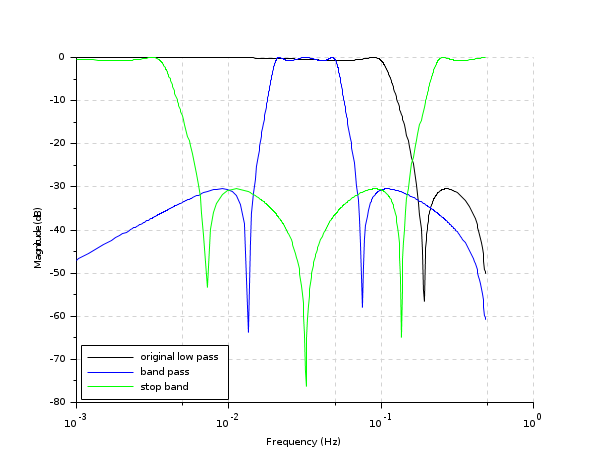Change language to:
English - Français - 日本語 - Русский

See the recommended documentation of this function

# trans

low-pass to other filter transform

### Syntax

```hzt=trans(hz,tr_type,frq)
hzt=trans(pd,zd,gd,tr_type,frq)
[pt,zt,gt]=trans(hz,tr_type,frq)
[pt,zt,gt]=trans(pd,zd,gd,tr_type,frq)```

### Arguments

hz

a single input single output discrete transfer function, the low pass filter

pd

Vector of given filter poles

zd

Vector of given filter zeros

gd

scalar: the given filter gain

tr_type

string, the type of transformation, see description for possible values

frq

2-vector of discrete cut-off frequencies (i.e.,`0<frq<.5`). see description for details.

hzt

transformed filter transfert function.

pt

vector of transformed filter zeros.

zt

vector of transformed filter poles.

gt

a scalar: transformed filter gain.

### Description

function for transforming standardized low-pass filter given its poles-zeros_gain representation into one of the following filters:

tr_type='lp'

low pass filter, the cutoff frequency is given by the first entry of `frq`, the second one is ignored.

tr_type='hp'

high pass filter, the cutoff frequency is given by the first entry of `frq`, the second one is ignored.

tr_type='bp'

band pass filter, the frequency range is given by `frq(1)` and `frq(2)`.

tr_type='sb'

stop band filter, the frequency range is given by `frq(1)` and `frq(2)`.

bilt

### Examples

```Hlp=iir(3,'lp','ellip',[0.1 0],[.08 .03]);
Hbp=trans(Hlp,'bp',[0.01 0.1]);
Hsb=trans(Hlp,'sb',[0.01 0.1])

clf();gainplot([Hlp;Hbp;Hsb],1d-3,0.48);
l=legend(['original low pass';'band pass';'stop band']);
l.legend_location="in_lower_left";```